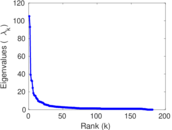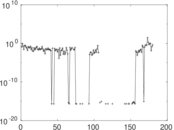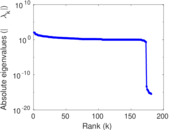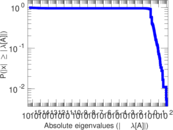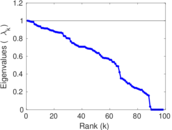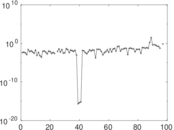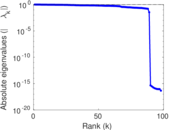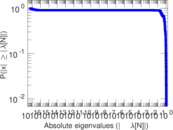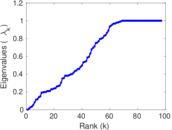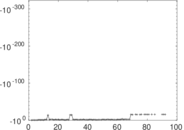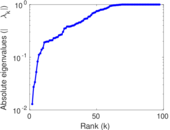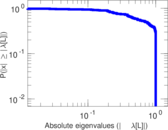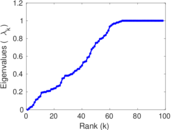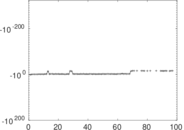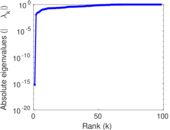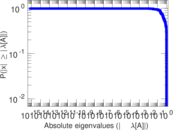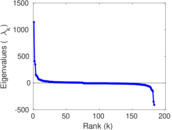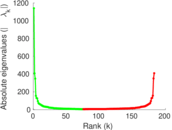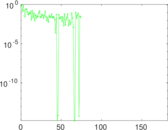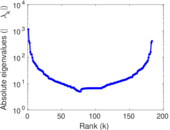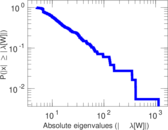# Wiktionary edits (iu)

This is the bipartite edit network of the Inuktitut Wiktionary. It contains users and pages from the Inuktitut Wiktionary, connected by edit events. Each edge represents an edit. The dataset includes the timestamp of each edit.

 Code `miu` Internal name `edit-iuwiktionary` Name Wiktionary edits (iu) Data source http://dumps.wikimedia.org/ AvailabilityDataset is available for download Consistency checkDataset passed all tests Category Authorship network Dataset timestamp 2017-10-20 Node meaning User, article Edge meaning Edit Network formatBipartite, undirected Edge typeUnweighted, multiple edges Temporal dataEdges are annotated with timestamps

## Statistics

 Size n = 1,152 Left size n1 = 183 Right size n2 = 969 Volume m = 4,910 Unique edge count m̿ = 2,432 Wedge count s = 155,979 Claw count z = 8,850,183 Cross count x = 424,464,789 Square count q = 173,931 4-Tour count T4 = 2,020,536 Maximum degree dmax = 780 Maximum left degree d1max = 780 Maximum right degree d2max = 44 Average degree d = 8.524 31 Average left degree d1 = 26.830 6 Average right degree d2 = 5.067 08 Fill p = 0.013 714 8 Average edge multiplicity m̃ = 2.018 91 Size of LCC N = 842 Diameter δ = 14 50-Percentile effective diameter δ0.5 = 4.380 02 90-Percentile effective diameter δ0.9 = 7.611 28 Median distance δM = 5 Mean distance δm = 4.992 25 Gini coefficient G = 0.757 900 Balanced inequality ratio P = 0.197 556 Left balanced inequality ratio P1 = 0.105 703 Right balanced inequality ratio P2 = 0.235 642 Relative edge distribution entropy Her = 0.810 293 Power law exponent γ = 2.548 55 Tail power law exponent γt = 1.921 00 Tail power law exponent with p γ3 = 1.921 00 p-value p = 0.000 00 Left tail power law exponent with p γ3,1 = 1.691 00 Left p-value p1 = 0.207 000 Right tail power law exponent with p γ3,2 = 8.471 00 Right p-value p2 = 0.234 000 Degree assortativity ρ = +0.216 985 Degree assortativity p-value pρ = 2.638 70 × 10−27 Spectral norm α = 105.088 Algebraic connectivity a = 0.012 894 7 Spectral separation |λ1[A] / λ2[A]| = 1.130 03 Controllability C = 796 Relative controllability Cr = 0.697 023

## Plots

### Fruchterman–Reingold graph drawing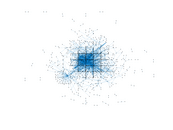### Degree distribution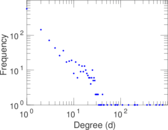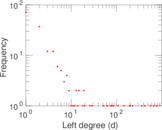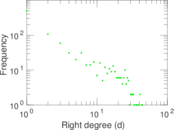### Cumulative degree distribution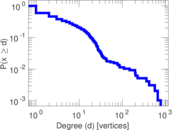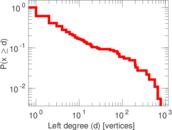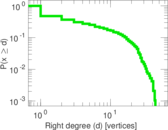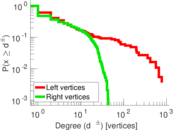### Lorenz curve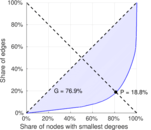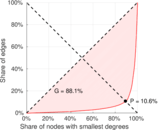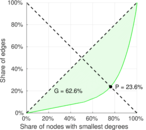### Spectral distribution of the adjacency matrix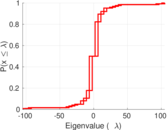### Spectral distribution of the normalized adjacency matrix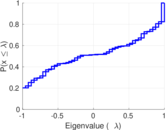### Spectral distribution of the Laplacian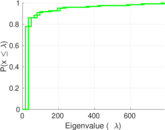### Spectral graph drawing based on the adjacency matrix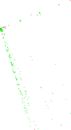### Spectral graph drawing based on the Laplacian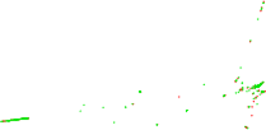### Spectral graph drawing based on the normalized adjacency matrix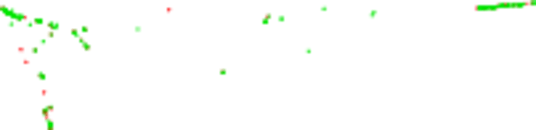### Degree assortativity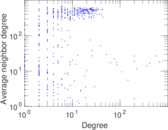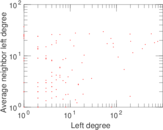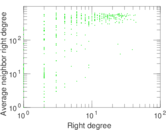### Zipf plot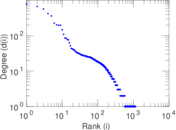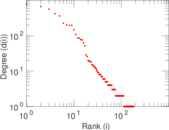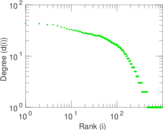### Hop distribution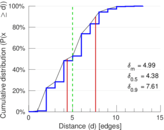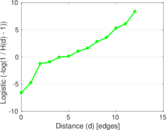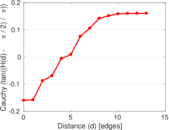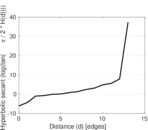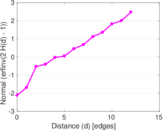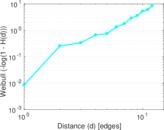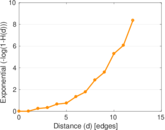### Double Laplacian graph drawing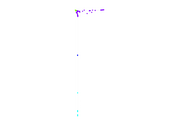### Delaunay graph drawing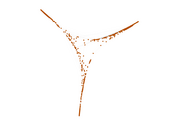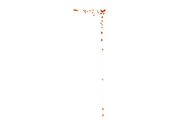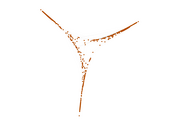### Edge weight/multiplicity distribution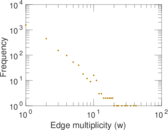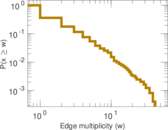### Temporal distribution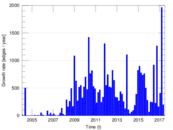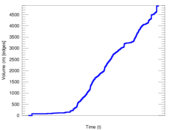### Temporal hop distribution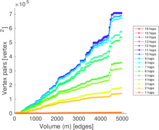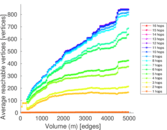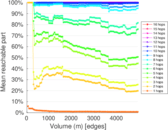### Diameter/density evolution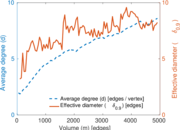### Matrix decompositions plots